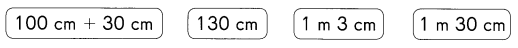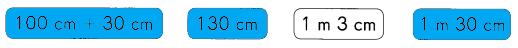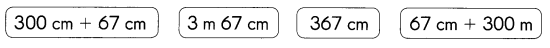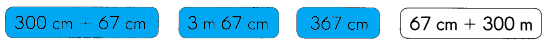# Math in Focus Grade 3 Chapter 11 Practice 1 Answer Key Meters and Centimeters

Practice the problems of Math in Focus Grade 3 Workbook Answer Key Chapter 11 Practice 1 Meters and Centimeters to score better marks in the exam.

## Math in Focus Grade 3 Chapter 11 Practice 1 Answer Key Meters and Centimeters

Complete.

Example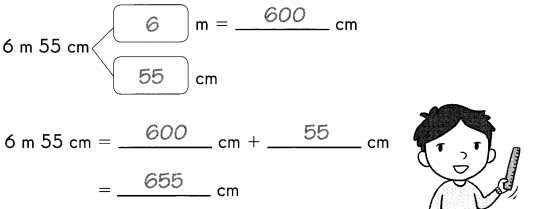Question 1.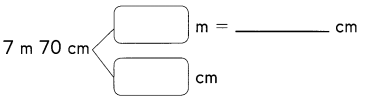7 m 70 cm = __________ cm + __________ cm
= ___________ cm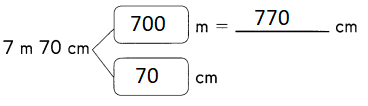Explanation:
Converting meter to centimeter
1 meter = 100 centimeter
7 m 70 cm = 700 cm + 70 cm
= 770 cm

Question 2.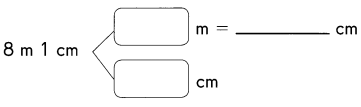8 m 1 cm = __________ cm + __________ cm
= ___________ cm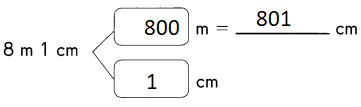Explanation:
Converting meter to centimeter
1 meter = 100 centimeter

Write in centimeters.

Question 3.
6 m 96 cm = ___________ cm + ___________ cm = ___________ cm
6 m 96 cm = 600 cm + 96 cm = 696 cm
Explanation:
Converting meter to centimeter
1 meter = 100 centimeter

Question 4.
8 m 90 cm = _________ cm + _________ cm = _________ cm
8 m 90 cm = 800 cm + 90 cm = 890 cm
Explanation:
Converting meter to centimeter
1 meter = 100 centimeter

Question 5.
9 m 20 cm = ___________ cm + __________ cm = __________ cm
9 m 20 cm = 900 cm + 20 cm = 920 cm
Explanation:
Converting meter to centimeter
1 meter = 100 centimeter

Question 6.
9 m 2 cm = ___________ cm + ___________ cm = ___________ cm
9 m 2 cm = 900 cm + 2 cm = 902 cm
Explanation:
Converting meter to centimeter
1 meter = 100 centimeter

Complete.

Example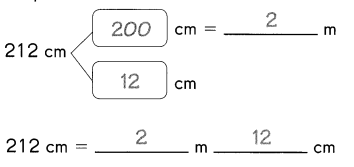Question 7.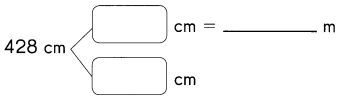428 cm = ___________ m ___________ cm

Question 8.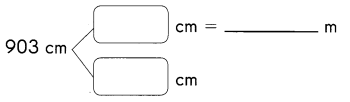903 cm = ___________ m ___________ cm

Write in meters and centimeters.

Question 9.
123 cm = ___________ cm + ___________cm = ___________ m ___________ cm
123 cm = 100 cm + 23 cm = 1 m 23 cm
Explanation:
Converting given  centimeter to meter  and centimeter
1 meter = 100 centimeter

Question 10.
390 cm = ___________ cm + ___________cm = ___________ m ___________ cm
390 cm = 300 cm + 90 cm = 3 m 90 cm
Explanation:
Converting given  centimeter to meter  and centimeter
1 meter = 100 centimeter

Question 11.
365 cm = ___________ cm + ___________cm = ___________ m ___________ cm
365 cm = 300 cm + 65 cm = 3 m 65 cm
Explanation:
Converting given  centimeter to meter  and centimeter
1 meter = 100 centimeter

Question 12.
909 cm = ___________ cm + ___________cm = ___________ m ___________ cm
909 cm = 900 cm + 9 cm = 9 m 9 cm
Explanation:
Converting given  centimeter to meter  and centimeter
1 meter = 100 centimeter

Choose the unit you would use to measure each. Write meters or centimeters.

Question 13.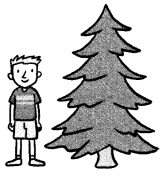This pine tree is about 2 _________________ tall.
This pine tree is about 2 meters tall.
Explanation:
The height of the tree will be more
we cannot measure in centimeters
so, it might be 2 meters

Question 14.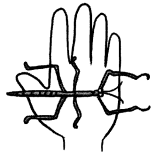The insect is about 10 _____________ long.
The insect is about 10 centimeters long.
Explanation:
The height of the Insects will be small
we cannot measure in meters
so, it might be 10 centimeters

Fill in the blanks. Color the banner with the longest measurement.

Example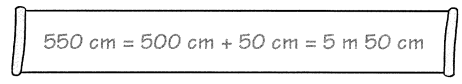Question 15.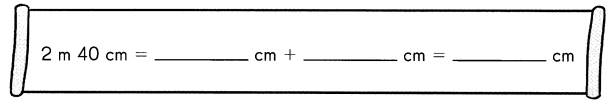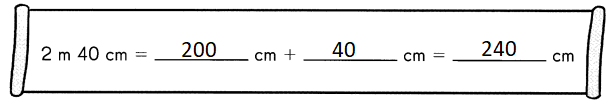Explanation:
Converting meter to centimeter
1 meter = 100 centimeter

Question 16.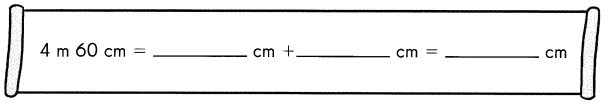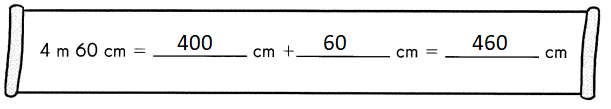Explanation:
Converting meter to centimeter
1 meter = 100 centimeter

Question 17.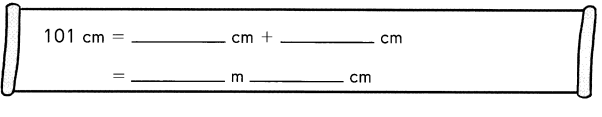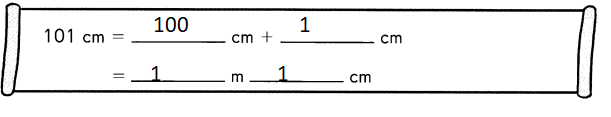Explanation:
Converting given  centimeter to meter  and centimeter
1 meter = 100 centimeter

Color the boxes with equal measurements.

Question 18.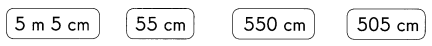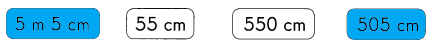Explanation:
1 meter = 100 cm
5 m 5cm = 500 + 5 = 505 cm

Question 19.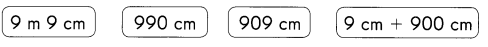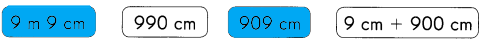Explanation:
1 meter = 100 cm
9 m 9 cm = 900 + 9 = 909 cm

Question 20.